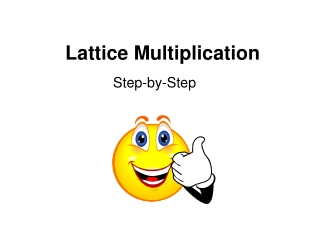DownloadDownload PresentationLattice Multiplication

# Lattice Multiplication

Download Presentation## Lattice Multiplication

- - - - - - - - - - - - - - - - - - - - - - - - - - - E N D - - - - - - - - - - - - - - - - - - - - - - - - - - -
##### Presentation Transcript

1. Lattice Multiplication Step-by-Step

2. 356 * 25 = I don’t remember how to multiply!!!

3. I can help!! Let me show you how to do Lattice Multiplication. HELP!!!

4. 356 * 25 Make a grid the exact size of your problem. A 2 digit number times a 3 digit number gets a 2 x 3 grid. Draw a diagonal line in each box. 5 3 6 2 5

5. 356 * 25 Multiply 6 * 2 and place the answer in the grid where the lines meet. 5 3 6 1 2 2 5

6. 356 * 25 Multiply 5 * 2 and place the answer in the grid where the lines meet. 5 3 6 1 1 2 0 2 5

7. 356 * 25 Multiply 3 * 2 and place the answer in the grid where the lines meet. Use a zero as a place holder. 5 3 6 0 1 1 2 0 6 2 5

8. 356 * 25 Multiply 6 * 5 and place the answer in the grid where the lines meet. 5 3 6 0 1 1 2 0 6 2 3 5 0

9. 356 * 25 Multiply 5 * 5 and place the answer in the grid where the lines meet. 5 3 6 0 1 1 2 0 6 2 2 3 5 5 0

10. 356 * 25 Multiply 3 * 5 and place the answer in the grid where the lines meet. 5 3 6 0 1 1 2 0 6 2 1 2 3 5 5 5 0

11. 356 * 25 Now add the diagonals and place the answer below the grid. Be sure to carry to the next diagonal if you have a 2 digit answer. 5 3 6 0 1 1 2 0 6 2 1 2 3 5 5 5 0 0

12. 356 * 25 Now add the diagonals and place the answer below the grid. Be sure to carry to the next diagonal if you have a 2 digit answer. 5 3 6 0 1 1 +1 2 0 6 2 1 2 3 5 5 5 0 0 0

13. 356 * 25 Now add the diagonals and place the answer below the grid. Be sure to carry to the next diagonal if you have a 2 digit answer. 5 3 6 0 1 1 +1 2 0 6 2 1 2 3 5 5 5 0 9 0 0

14. 356 * 25 Now add the diagonals and place the answer below the grid. Be sure to carry to the next diagonal if you have a 2 digit answer. 5 3 6 0 1 1 +1 2 0 6 2 1 2 3 5 8 5 5 0 9 0 0

15. 356 * 25 Now add the diagonals and place the answer below the grid. Be sure to carry to the next diagonal if you have a 2 digit answer. 5 3 6 0 1 1 +1 2 0 6 2 1 2 3 5 8 5 5 0 9 0 0

16. That is Soooo Cool!!! Thanks! 356 * 25 = 8,900